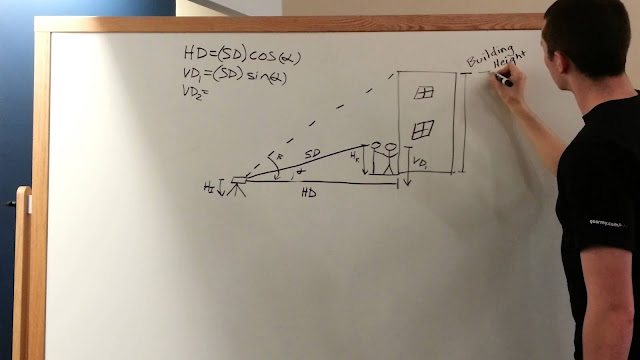# Engineering Society

Process for finding the height of a tall object using a total station and prism rod. let me tell you let me show you through narrati...

# Learn How to Determine Height of a Building Using Total StationProcess for finding the height of a tall object using a total station and prism rod.
let me tell you let me show you through narration I'm all yours the first thing you're going to have to do is properly set up your total station which you can see demonstrated in another one of our educational videos

the next step is to measure the height of the instrument and the height of your rod you can do so using a measuring tape or a measuring stick once your instrument is set up you aim the total station at the prism at the top of your rod with this the total station will read to you your slope distance and your angle alpha after you tube this you aim your total station at the top corner of the building it will now read to you your new angle beta these are all the readings you need to make the calculations to determine the height of your building

the next step would be to find your horizontal distance to find your horizontal distance is the slope distance times the cosine of alpha

the next step is to calculate the vertical distance one this is determined by multiplying the slope distance times the sine of alpha after this you need to determine the vertical distance to and this distance is determined by multiplying your horizontal distance by the tangent of beta but these you can get your building height the height is simply vertical distance two - vertical distance one plus the height of your rod that's all we have for the building height tune.